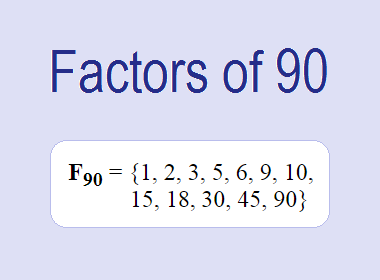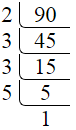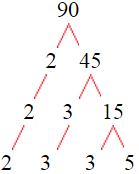# Factors of 90The factors of 90 are 1, 2, 3, 5, 6, 9, 10, 15, 18, 30, 45, and 90 i.e. F90 = {1, 2, 3, 5, 6, 9, 10, 15, 18, 30, 45, 90}. The factors of 90 are those numbers that can divide 90 without leaving a remainder.

We can check if these numbers are factors of 90 by dividing 90 by each of them. If the result is a whole number, then the number is a factor of 90. Let's do this for each of the numbers listed above:

·        1 is a factor of 90 because 90 divided by 1 is 90.

·        2 is a factor of 90 because 90 divided by 2 is 45.

·        3 is a factor of 90 because 90 divided by 3 is 30.

·        5 is a factor of 90 because 90 divided by 5 is 18.

·        6 is a factor of 90 because 90 divided by 6 is 15.

·        9 is a factor of 90 because 90 divided by 9 is 10.

·        10 is a factor of 90 because 90 divided by 10 is 9.

·        15 is a factor of 90 because 90 divided by 15 is 6.

·        18 is a factor of 90 because 90 divided by 18 is 5.

·        30 is a factor of 90 because 90 divided by 30 is 3.

·        45 is a factor of 90 because 90 divided by 45 is 2.

·        90 is a factor of 90 because 90 divided by 90 is 1.

## How to Find Factors of 90?

1 and the number itself are the factors of every number. So, 1 and 90 are two factors of 90. To find the other factors of 90, we can start by dividing 90 by the numbers between 1 and 90. If we divide 90 by 2, we get a remainder of 0. Therefore, 2 is a factor of 90. If we divide 90 by 3, we get a remainder of 0. Therefore, 3 is also a factor of 90.

Next, we can check if 4 is a factor of 90. If we divide 90 by 4, we get a remainder of 2. Therefore, 4 is not a factor of 90. We can continue this process for all the possible factors of 90.

Through this process, we can find that the factors of 90 are 1, 2, 3, 5, 6, 9, 10, 15, 18, 30, 45, and 90. These are the only numbers that can divide 90 without leaving a remainder.********************

********************

## Properties of the Factors of 90

The factors of 90 have some interesting properties. One of the properties is that the sum of the factors of 90 is equal to 234. We can see this by adding all the factors of 90 together:

1 + 2 + 3 + 5 + 6 + 9 + 10 + 15 + 18 + 30 + 45 + 90 = 234

Another property of the factors of 90 is that they are all composite numbers except 1, 2, 3, and 5.

## Applications of the Factors of 90

The factors of 90 have several applications in mathematics. One of the applications is in finding the highest common factor (HCF) of two or more numbers. The HCF is the largest factor that two or more numbers have in common. For example, to find the HCF of 90 and 120, we need to find the factors of both numbers and identify the largest factor they have in common. The factors of 90 are 1, 2, 3, 5, 6, 9, 10, 15, 18, 30, 45, and 90. The factors of 120 are 1, 2, 3, 5, 6, 8, 10, 12, 15, 20, 24, 30, 40, 60, and 120. The largest factor that they have in common is 30. Therefore, the HCF of 90 and 120 is 30.

Another application of the factors of 90 is in prime factorization. Prime factorization is the process of expressing a number as the product of its prime factors. The prime factors of 90 are 2, 3, and 5, since these are the only prime numbers that can divide 90 without leaving a remainder. Therefore, we can express 90 as:

90 = 2 × 3 × 3 × 5

We can do prime factorization by division and factor tree method also. Here is the prime factorization of 90 by division method,90 = 2 × 3 × 3 × 5

Here is the prime factorization of 90 by the factor tree method,90 = 2 × 3 × 3 × 5

## Conclusion

The factors of 90 are the numbers that can divide 90 without leaving a remainder. The factors of 90 are 1, 2, 3, 5, 6, 9, 10, 15, 18, 30, 45, and 90. The factors of 90 have some interesting properties, such as having a sum of 234. The factors of 90 have several applications in mathematics, such as finding the highest common factor and prime factorization.